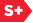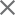### Proceedings Paper

A linear programming based algorithm for determining corresponding point tuples in multiple vascular images
Author(s): Vikas Singh; Jinhui Xu; Kenneth R. Hoffmann; Peter B. Noël; Alan M. Walczak
Format Member Price Non-Member Price
PDF \$17.00 \$21.00

Paper Abstract

Multi-view imaging is the primary modality for high-spatial-resolution imaging of the vasculature. The 3D vascular structure can be reconstructed if the imaging geometries are determined using known corresponding point-pairs (or k-tuples) in two or more images. Because the accuracy improves with more input corresponding point-pairs, we propose a new technique to automatically determine corresponding point-pairs in multi-view (k-view) images, from 2D vessel image centerlines. We formulate the problem, first as a multi-partite graph-matching problem. Each 2D centerline point is a vertex; each individual graph contains all vessel-points (vertices) in an image. The weight ('cost') of the edges between vertices (in different graphs) is the shortest distance between the points' respective projection-lines. Using this construction, a universe of mappings (k-tuples) is created, each k-tuple having k vertices (one from each image). A k-tuple's weight is the sum of pair-wise 'costs' of its members. Ideally, a set of such mappings is desired that preserves the ordering of points along the vessel and minimizes an appropriate global cost function, such that all vertices (in all graphs) participate in at least one mapping. We formulate this problem as a special case of the well-studied Set-Cover problem with additional constraints. Then, the equivalent linear program is solved, and randomized-rounding techniques are used to yield a feasible set of mappings. Our algorithm is efficient and yields a theoretical quality guarantee. In simulations, the correct matching is achieved in ~98% cases, even with high input error. In clinical data, apparently correct matching is achieved in >90% cases. This method should provide the basis for improving the calculated 3D vasculature from multi-view data-sets.

Paper Details

Date Published: 10 March 2006
PDF: 12 pages
Proc. SPIE 6144, Medical Imaging 2006: Image Processing, 61442D (10 March 2006); doi: 10.1117/12.651707
Show Author Affiliations
Vikas Singh, SUNY at Univ. of Buffalo (United States)
Jinhui Xu, SUNY at Univ. of Buffalo (United States)
Kenneth R. Hoffmann, SUNY at Univ. of Buffalo (United States)
Peter B. Noël, SUNY at Univ. of Buffalo (United States)
Alan M. Walczak, SUNY at Univ. of Buffalo (United States)

Published in SPIE Proceedings Vol. 6144:
Medical Imaging 2006: Image Processing
Joseph M. Reinhardt; Josien P. W. Pluim, Editor(s)